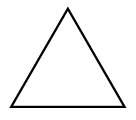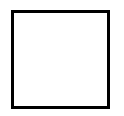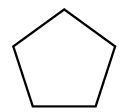### Home > MC1 > Chapter 1 > Lesson 1.1.2 > Problem1-13

1-13.

Consider the first three figures of the pattern below.Figure 1Figure 2Figure 3

1. On your own paper, draw what Figure 4 of this pattern should look like.

How many sides are in each figure shown?
How many would you expect in the next figure?

2. Using words, describe what Figures 5 and 6 should look like.

If Figure 4 has $6$ sides of the same length, how many sides should the next figures have?

Figure 5 should have seven sides of the same length.
Figure 6 should have eight sides of the same length.